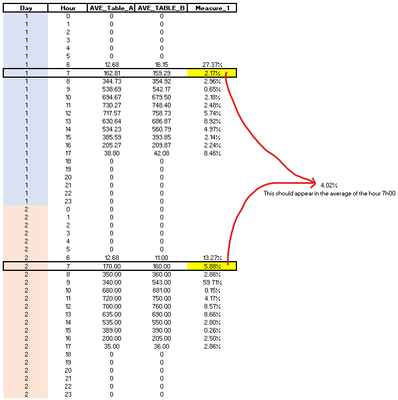cancel
Showing results for
Did you mean:Frequent Visitor

## Calculate a measure of each step and then do the average of that result

Hello,

I have two Data tables (Data_table_A & Data_table_B). Both of them are connected using two relations with two fact tables (Fact_table_Date & Fact_table_Time).I did a visual table to calculate the average error filtering by hour, using a Measure_1:However, I saw my Measure_1 is not working on the way I need. As you can see, my results on the column Measure_1 is the error for the average I calculated in the columns AVE_table_A & AVE_table_B. Even it calculates the error for the totals (Blue), when my average for that column is different (green).

My code:

Measure_! =
//Calculates average by hour
VAR _Actual = [AVE_table_A]
VAR _Forecast = [AVE_table_B]

//Calculates the differences between both values
VAR _Error = ABS(_Actual - _Forecast)

//Return the error if the data is not below zero or is empty
RETURN
AVERAGEX(
VALUES('Fact Table_TIME'[Hour]),
IF(
OR(Actual <= 0, Forecast <= 0),
BLANK(),
DIVIDE(_Error, Actual)
)
)

What I try to do is :
1. Go to each hour on each day, calculate the average of each table (Because each table have data for each minute), then calculate the error for that hour
2. Then, do the average of each error on each hour
3. In the final table show the average of the column and not calculate the measure.

Example of the calculation only for two day (Normally I will do it for each month):P.D: I read this article which explain why I have that result at the end of my table. I understand it. But I require the average on my table, not the other result.

1 ACCEPTED SOLUTIONSuper User

Hello again @Bullpro_

Measure_! =
AVERAGEX (
CROSSJOIN (
VALUES ( 'Fact Table_DATE'[Date] ),
VALUES ( 'Fact Table_TIME'[Hour] )
),
//Calculates average by hour
VAR _Actual = [AVE_table_A]
VAR _Forecast = [AVE_table_B] //Calculates the differences between both values
VAR _Error =
ABS ( _Actual - _Forecast ) //Return the error if the data is not below zero or is empty
RETURN
IF ( OR ( Actual <= 0, Forecast <= 0 ), BLANK (), DIVIDE ( _Error, Actual ) )
)

3 REPLIES 3Super User

Hello again @Bullpro_

Measure_! =
AVERAGEX (
CROSSJOIN (
VALUES ( 'Fact Table_DATE'[Date] ),
VALUES ( 'Fact Table_TIME'[Hour] )
),
//Calculates average by hour
VAR _Actual = [AVE_table_A]
VAR _Forecast = [AVE_table_B] //Calculates the differences between both values
VAR _Error =
ABS ( _Actual - _Forecast ) //Return the error if the data is not below zero or is empty
RETURN
IF ( OR ( Actual <= 0, Forecast <= 0 ), BLANK (), DIVIDE ( _Error, Actual ) )
)Frequent Visitor

Thank you again @tamerj1 , It works perfectly.

Let ask again. Can you just explain why the order impacts directly in this case and why we should use the function CROSSJOIN?

Thank you!Super User

Because you have two fact tables the iteration is required across the crossjoin of the columns of dimension tables. This simulates your table visual